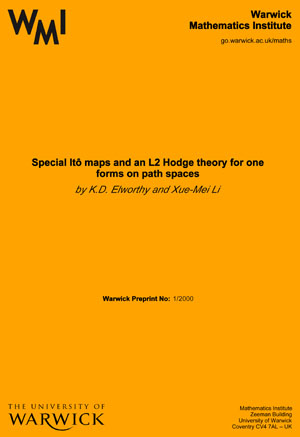# WMI Preprints 1978

Injective hulls of group rings,
by K.A. Brown & J. Lawrence

The invertible ideal theorem for right Noetherian rings,
by A.W. Chatters, A.W. Goldie, C.R. Hajarnavis & T.H. Lenagan

by E.C. de Sa

Unique ergodicity for quasi-invariant measures,
by K. Schmidt

Soluble groups with coherent group ring,
by R. Bieri & R. Strebel

A class of probability measures on groups arising from some problems in ergodic theory,
by V. Losert & K. Schmidt

Coboundaries and homomorphisms for nonsingular actions and a problem by H. Helson,
by C.C. Moore & K. Schmidt

Notes on the associated graded ring of a group ring,
by I.B.S. Passi

On the local stability of wave fronts defined by eikonal equations,
by D. Rand

Modular representations of finite groups with unsaturated split (B,N)-pairs,
by N.B. Tinberg

Augmentation quotients of some nonabelian finite groups,
by G. Losey & N. Losey

Some indecomposable modules of groups with split (B,N)-pairs,
by N.B. Tinberg

K-theory and stable structure of Noetherian group rings,
by K.A. Brown, T.H. Lenagan & J.T. Stafford

On the classifying spaces of discrete monoids,
by D. McDuff

Remarks on the GL2 of a ring,
by P. Menal

Nonlinear modeling of multistable perception,
by T. Poston & I. Stewart

On the sequence entropy of transformations with quasi discrete spectrum,
by P. Hulse

A lower bound for the entropy of certain maps of the unit intervals,
by L. Jonker & D. Rand

The stable structure of the augmentation quotients of the groups of the order p3,
by G. Losey & N. Losey

The homology of some groups of diffeomorphisms,
by D. McDuff

Finitary isomorphisms with finite expected code lengths,
by W. Parry

Bifurcations in one dimension. II. A versal model for bifurcations,
by L. Jonker & D. Rand

On the surfaces of Delaunay and their Gauss maps,
by J. Eells

Ratio in early Greek mathematics,
by D.H. Fowler

Approximations and the spectral properties of measure-preserving group actions,
by G.W. Riley

On the ergodicity of some real line extensions of irrational rotations,
by G.W. Riley & G. Atkinson

An infinite dimensional generalisation of the Cameron-Martin-Girsanov theorem,
by D. Bell

Prime ends,
by D.B.A. Epstein

The geometry of Mahowald orientations,
by B.J. Sanderson

Markov maps associated with Fuchsian groups,
by R. Bowen & C. Series

Applications and invocations of catastrophe theory,
by I. Stewart

Homotopy equivalences of manifolds,
by M. Weiss

Bifurcations on one dimension I. The nonwandering set,
by L. Jonker & D. Rand

Torsion products as homotopy groups,
by C.A. Robinson

Asymptotically invariant sequences and an action of SL(2,Z) on the 2-sphere,
by K. Schmidt

Graphical representation of n-dimensional space groups,
by R.L.E. Schwarzenberger

Pointwise periodic homeomorphisms,
by D.B.A. Epstein

Injective modules for groups rings of polycyclic groups I,
by I.M. Musson

Mixing properties of linear mod 1 maps of the unit interval
by M.R. Palmer

### Search WMI Preprint Catalogue: